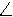Name _____________________________Date ___________________
Angles (Parallelogram and Rhombus)

 1 In parallelogram JBCK, the difference in the measure of two consecutive angles is 10. What is the degree measure of the smaller angle?
 2 In rhombus WCAG, AG = x + 59 and CA = 6x - 61. What is the perimeter of WCAG?
 3 In parallelogram SZNU, a diagonal is drawn from Z to U and the mNZU = 72, what is the mN?
 4 In parallelogram ABCD, mA = x + 18 and mC = 2x - 4. What is the value of x?
 5 In rhombus RAQJ, QJ = x + 39 and AQ = x + 39. What is the value of x?
 6 In parallelogram ENXY, mY = 64, what is the mE?
 7 In parallelogram ABCD, mC = 2x - 1 and mD = 1 + 8x. What is the value of x?
 8 In parallelogram CSDW, a diagonal is drawn from S to W and the mDSW = 21, what is the mW?
 9 In rhombus GBOY, BO = 88 + x and GB = 13x - 20. What is the value of x?
 10 In rhombus UGIY, IY = 6x - 72 and UG = 2x + 16. What is the perimeter of UGIY?
 11 In parallelogram OWFI, mO = 49, what is the mF?
 12 In parallelogram CDEO, the difference in the measure of two consecutive angles is 76. What is the degree measure of the larger angle?
 13 In parallelogram ABCD, mA = x + 86 and mB = 46 + 11x. What is the value of x?
 14 In parallelogram ABCD, mB = 7x - 25 and mD = 5 + 5x. What is the value of x?
 15 In parallelogram YTHE, the difference in the measure of two consecutive angles is 52. What is the degree measure of the smaller angle?
 16 In parallelogram BEIR, mI = 121, what is the mB?